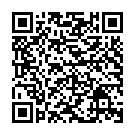## Subtraction Using a Number Line

Practice jumping from the smaller number to the larger number on a number line. Lots of choice of level, including; jumping from a 2-digit number to 100, from a 2-digit number to a 3-digit number and between 3-digit numbers. Great for developing mental methods of calculation.Scan to open this game on a mobile device. Right-click to copy and paste it onto a homework sheet.

### Game Objectives

New Maths Curriculum:

Year 2: Add and subtract numbers using concrete objects, pictorial representations, and mentally, including: a two-digit number and ones; a two-digit number and tens; two two-digit numbers;adding three one-digit numbers

Year 3: Add and subtract numbers mentally, including: a three-digit number and ones; a three-digit number and tens; a three-digit number and hundreds

KS2 Primary Framework:

Year 3:

Add or subtract mentally combinations of one–digit and two–digit numbers (Block A and D)

Develop and use written methods to record, support or explain addition and subtraction of two–digit and three–digit numbers (Block A and E)

Represent the information in a puzzle or problem using numbers, images or diagrams; use these to find a solution and present it in context (Block B)